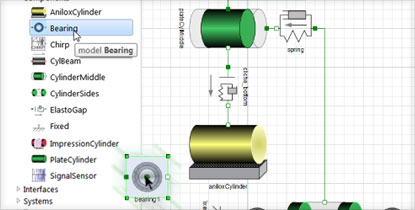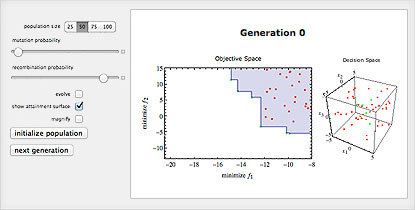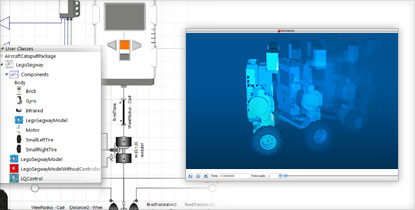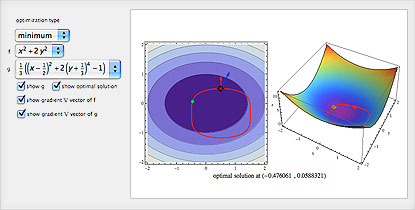# The Wolfram Solution for Industrial Engineering

Optimize your processes, implement quality management measures, rapidly prototype and deploy interactive applications and generate live reports automatically—all in one system, with one integrated workflow.

Underlying the Wolfram industrial engineering solution are state-of-the-art algorithms; sophisticated statistical analysis tools; self-checking, high-precision arithmetic; and the world's most accurate symbolic and numeric engine.

• The Wolfram Edge
• How Wolfram Compares
• Key Capabilities

Wolfram technologies include thousands of built-in functions that let you:

• Design and simulate manufacturing processes with Wolfram SystemModeler
• Develop statistical process control measures
• Design and analyze supply chains
• Design custom engineering components that adhere to tight tolerances
• Develop computer simulations of industrial engineering systems
• Improve manufacturing process efficiencies using lean manufacturing techniques
• Implement quality management methodologies, such as Six Sigma
• Develop production schedules and establish inventory levels
• Automatically compute design quantities, including closed-loop transfer functions, PID parametrizations and more
• Predict failure of componentsAnalyzing configurations in which cylinder bounce occurs in a printing press with Wolfram SystemModelerFinding the solution to an evolutionary multi-objective optimization problem interactively

Does your current tool set have these advantages?

• Built-in functionality for constrained and unconstrained optimization, statistical analysis and computation, simulation, curve fitting and a range of other application areas
Matlab requires the purchase of multiple toolboxes
• Instant, interactive interface construction using a single command to visualize your simulations, examine prototype sensitivity to parameter changes and more
Unique to Wolfram technologies
• Easy-to-use parallel computing capabilities for solving computation- or data-intensive problems on multicore computers or grids
Extensive programming is required to parallelize processes in all other systems
Matlab requires an extra-cost toolbox
• Automated precision control and arbitrary-precision numerics produce accurate results for large-scale finite element analysis and other problems
Matlab relies on finite-precision numerics, which can cause serious errors due to lack of precision
• Highly optimized superfunctions analyze your equations and automatically select the right algorithms to get you accurate results quickly—sometimes switching mid-calculation for further optimization
Non-Mathematica computation systems make you analyze your equations manually to determine which function to apply—for example, where in Mathematica you use NDSolve, in Matlab you must correctly choose among ode45, ode23, ode113, ode15s, bvp4c, pdepe and so on or risk wrong answers
• Complete workflow, from simulation to analysis to typeset document or interactive slide show, in a single document
Unique to Wolfram technologiesControlling an inverted pendulum with Wolfram SystemModelerSolving a constrained optimization problem

Wolfram SystemModeler is the most complete physical modeling and simulation tool for high-fidelity modeling. With SystemModeler, you can:

# Consulting Solutions

Enlist the world’s computation experts to help with your project—any size, any level. At Wolfram, we know what’s possible with computational technology because we are global leaders in creating it. That gives us an unprecedented depth of expertise in applying it to consulting work in a variety of fields. Whether individual or enterprise, from concept to deployment, our computation experts can help you achieve robust results with less time and effort. Get us started with your project today